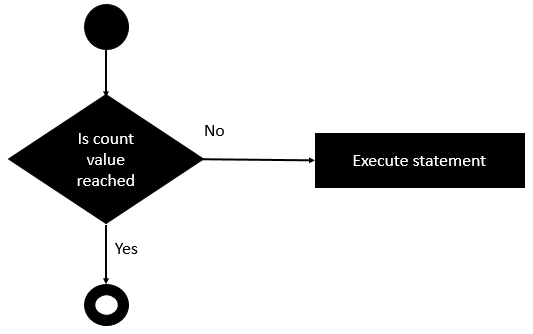# Rexx - do-until Loop

The do-until loop is a slight variation of the do while loop. This loop varies in the fact that is exits when the condition being evaluated is false.

## Syntax

The syntax of the do-until statement is as follows −

```do until (condition)
statement #1
statement #2
...
end
```

The do-until statement is different from the do-while statement in the fact, that it will only execute the statements until the condition evaluated is true. If the condition is true, then the loop is exited.

## Flow Diagram

The following diagram shows the diagrammatic explanation of this loop.The key thing to note is that the code block runs till the condition in the do-until evaluates to false. As soon as the condition evaluates to true, the do loop exits.

The following program is an example of a do-until loop statement.

## Example

```/* Main program */
j = 1

do until (j <= 10)
say j
j = j + 1
end
```

The output of the above code will be −

```1
```
rexx_loops.htm